# Test: Z-Transform - 1

## 10 Questions MCQ Test Signals and Systems | Test: Z-Transform - 1

Description
Attempt Test: Z-Transform - 1 | 10 questions in 30 minutes | Mock test for Electrical Engineering (EE) preparation | Free important questions MCQ to study Signals and Systems for Electrical Engineering (EE) Exam | Download free PDF with solutions
QUESTION: 1

###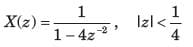Solution: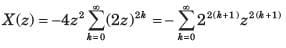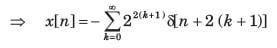QUESTION: 2

### X (z) = ln (1 + z-1 ), |z|>0

Solution: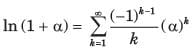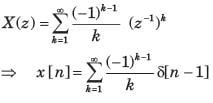QUESTION: 3

### If z-transform is given by X (z) = cos ( z -3), |z|> 0, The value of x is

Solution: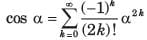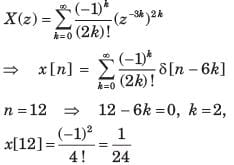QUESTION: 4

X (z) of a system is specified by a pole zero pattern in fig. P.5.4.36.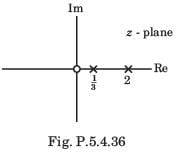Consider three different solution of x[n]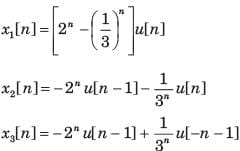Correct solution is

Solution:

All gives the same z transform with different ROC. So all are the solution.

QUESTION: 5

Consider three different signal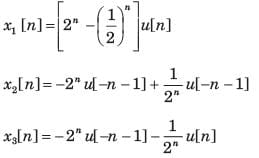Fig. P.5.4.37 shows the three different region. Choose the correct option for the ROC of signal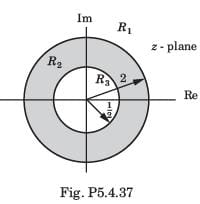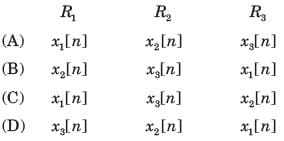Solution:

x1[n] is right-sided signal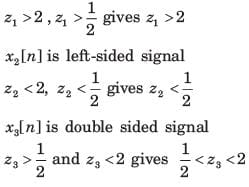QUESTION: 6

Given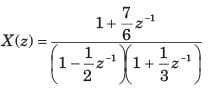For three different ROC consider there different solution of signal x[n] :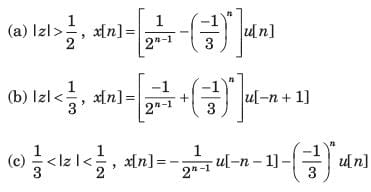Correct solutions are

Solution: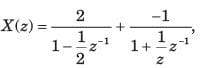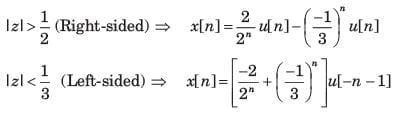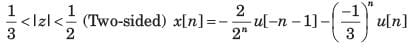So (b) is wrong.

QUESTION: 7

X (z) has poles at z = 1/2 and z = -1. If x  = 1 x [ -1] = 1, and the ROC includes the point z = 3/4. The time signal x[n] is

Solution:

Since the ROC includes the Z = 3/4, ROC is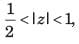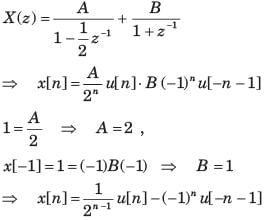QUESTION: 8

x[n] is right-sided, X (z) has a signal pole, and x = 2, x = 1/2. x[n] is

Solution:

x[n]= Cpnu[n],x[0=2=C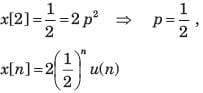QUESTION: 9

Find the Z-transform of y(n) = x(n + 2)u(n).

Solution:

Given y(n) = x(n + 2)u(n)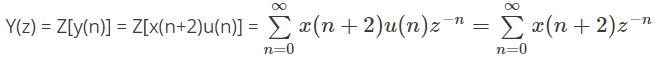Let n + 2 = p,i.e. n = p – 2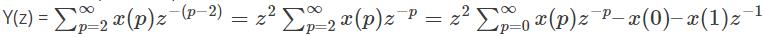=z2 X(Z) – z2 x(0) – zx(1).

QUESTION: 10

Let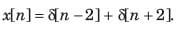The unilateral z-transform is

Solution: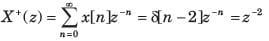Use Code STAYHOME200 and get INR 200 additional OFF Use Coupon Code